Courses

# NCERT Solutions - Stars and the Solar System, Science, Class 8 Class 8 Notes | EduRev

## Class 8 : NCERT Solutions - Stars and the Solar System, Science, Class 8 Class 8 Notes | EduRev

The document NCERT Solutions - Stars and the Solar System, Science, Class 8 Class 8 Notes | EduRev is a part of the Class 8 Course Class 8 Science by VP Classes.
All you need of Class 8 at this link: Class 8

NCERT TEXTBOOK QUESTIONS SOLVED

Choose the Correct Answer in Questions 1-3.

Q. 1. Which of the following is NOT a member of the solar system?

(a) An asteroid
(b) A satellite
(c) A constellation
(d) A comet.

Ans. (c) A constellation

Q. 2. Which of the following is NOT a planet of the sun?

(a) Sirius
(b) Mercury
(c) Saturn
(d) Earth

Ans. (a) Sirius

Q. 3. Phases of the moon occur because:
(a) we can see only that part of the moon which reflects light towards us.
(b) our distance from the moon keeps changing.
(c) the shadow of the earth covers only a part of the moon’s surface.
(d) the thickness of the moon’s atmosphere is not constant.
Ans.
(a) We can see only that part of the moon which reflects light towards us.

Q. 4. Fill in the blanks: (a) The planet which is farthest from the Sun is ______.
(b) The planet which appears reddish in colour is ______.
(c) A group of stars that appear to form a pattern in the sky is known as a ______.
(d) A celestial body that revolves around a planet is known as ______.
(e) Shooting stars are actually not ______.
(f) Asteroids are found between the orbits of ______ and ______.
Ans.
(a) Neptune
(b) Mars
(c) Constellation
(d) Satellite
(e) meteors
(f) Mars, Jupiter.

Q. 5. Mark the following statements as true or false:
(a) Pole star is a member of the solar system.
(b) Mercury is the smallest planet of the solar system.
(c) Uranus is the farthest planet in the solar system.
(d) INSAT is an artificial satellite.
(e) There are nine planets in the solar system.
(f ) Constellation Orion can be seen only with a telescope.
Ans.
(a) False
(b) True
(c) False
(d) True
(e) False
(f) False

Q. 6. Match items in Column A with one or more items in Column B:

 Column A Column B Inner planets Outer planets Constellation Satellite of the earth Saturn Pole star Great Bear Moon Earth Orion Mars

Ans.

 Column A Column B Inner planets Outer planets Constellation Satellite of the earth (g) Mars and (e) Earth (a) Saturn (c) Great Bear and (f) Orion (d) Moon

Q. 7. In which part of the sky can you find Venus, if it is visible as an evening star?
Ans
. In west side of the sky.

Q. 8. Name the largest planet of the solar system.
Ans.
The largest planet of the solar system is Jupiter.

Q. 9. What is constellation? Name any two constellations.
Ans.
A group of star which has a recognizable shape is called constellation. Two constellations are great Bear and Orion.

Q. 10. Draw sketches to show the relative positions of prominent stars in (a) Ursa Major (b) Orion.
Ans.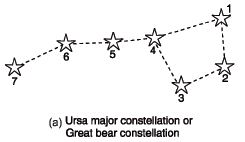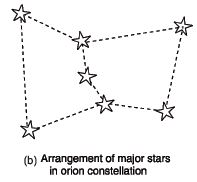Q. 11. Name two objects other than planets which are members of the solar system.
Ans.
Comets and asteroids.

Q . 12. Explain how you can locate the Pole star with the help of Ursa Major.
Ans.
Pole star can be located with the help of the three stars at the end of Ursa Major. Imagine a straight line passing through these stars. Extend the imaginary line in north direction. This line is about five times the distance between two stars. A star seen in this direction is the Pole star.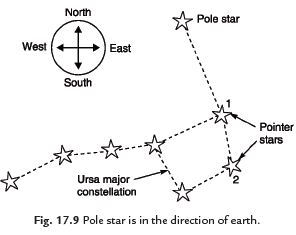Q . 13. Do all the stars in the sky move? Explain.
Ans.
No, all the stars do not move in the sky. They appear to move from east to west. It is due to the rotation of the earth on which we live. The earth moves from west to east. But Pole star does not appear to move.

Q. 14. Why is the distance between stars expressed in light years? What do you understand by the statement that a star is eight light years away from the earth?
Ans.
Stars are far away from each other. The distance between two stars is millions of kilometres. The distance between the sun and the earth is 150,000,000 km, whereas the distance of alpha centaury is 40,000,000,000,000 km. It is not convenient to show this distance in km. So it is expressed in light year. A light year is the distance covered by light in one year. Eight light year means the distance covered by light in eight years.

Q. 15. The radius of Jupiter is 11 times the radius of the Earth. Calculate the ratio of the volumes of Jupiter and the Earth. How many Earths can Jupiter accommodate?
Ans.
Let the radius of the Earth = R units

∴ Volume of the Earth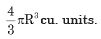Now, the radius of Jupiter = 11R units.

∴ Volume of Jupiter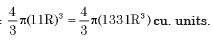Now the ratios of the volume of Jupiter and the Earth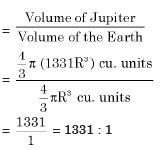So 1331 Earths can be accommodated in one Jupiter.

Q. 16. Boojho made the following sketch of the solar system (Fig. 17.10). Is the sketch correct? If not, correct it.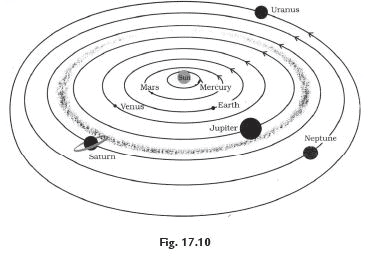Ans. No, the given sketch is not correct. The following figure is correct.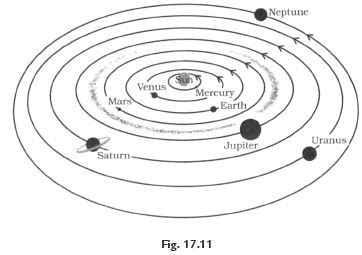Offer running on EduRev: Apply code STAYHOME200 to get INR 200 off on our premium plan EduRev Infinity!

90 docs|18 tests

,

,

,

,

,

,

,

,

,

,

,

,

,

,

,

,

,

,

,

,

,

,

,

,

,

,

,

;